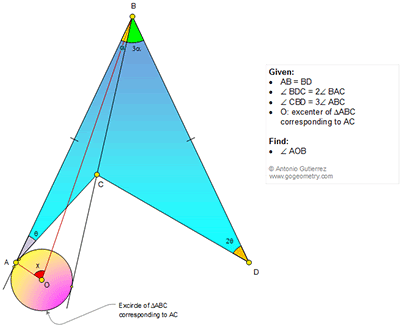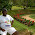## Saturday, December 26, 2015

### Geometry Problem 1174: Triangle, Quadrilateral, Double, Triple, Angle, Congruence, Excenter, Angle Bisector

Geometry Problem. Post your solution in the comments box below.
Level: Mathematics Education, High School, Honors Geometry, College.

Click the figure below to view more details of problem 1174.1.Let the bisector of < ABC meet AC at E, CD at F and AD at G.
For ease of typing I use @ for alpha and \$ for theta

Easily < ABO = OBC = @/2 and < CBE = @ so that < EBD = 2@
Also since BG is the perpendicular bisector of AD, < BAE = EAF = BDE = EDF = \$
So C is the incentre of Tr. ABF so < DFG = < AFG = < AFC = < CFE and each of these must therefore be essentially 60 degrees since the last 3 of these 4 angles add upto 180.
Hence from Tr. BDF, 2(&+\$) = 60 and so
&+\$ = 30.....(1)

In Tr. ABC, OA is an external angle bisector at A so x+@/2 = 90 -\$/2 from which
x + (@+\$)/2 = 90
Substituting @+& = 30 from (1) therefore
x+30/2 = 90 and hence x = 75

Sumith Peiris
Moratuwa
Sri Lanka

2.http://s17.postimg.org/59873mej3/pro_1174.png

Let angle bisector of angle ABD meet CD at F and AD at E
Observe that due to symmetry we have
∠(BAF)= ∠ (BDF)=2. Theta
And tri. AFD is isosceles
AC and BC are angles bisectors of ∠ (BAC) and ∠ (CFA)
So ∠ (BFC)= ∠ (CFA)=2.alpha+2.theta => ∠ (FDA)=alpha+theta
In right tri. BED we have alpha+theta=30
We have∠ (HOA)= theta/2 and ∠ (ABO)=alpha/2
In right tri. HBO we have x+theta/2+alpha/2= 90 => x= 75

3.Join AD and form the isosceles triangle ABD
E be foot of the perpendicular from B to AD
Say P be the point of intersection of BE and DC
Join AP and since ABD is isosceles m(CAP)=m(BAC)=θ and also APD is isosceles
=> C is the incenter of triangle ABP ----------(1)
A bit of angle chasing leads us to the below
m(BPC)=m(EPD)=2(α+θ) -----(2)
m(APC)=m(PAB)+m(PBA)=180-4(α+θ) -----------(3)

From (1), we have (2)=(3)
=>(α+θ)=30 ----------(4)
Now consider the triangle BAX
we have X=180-(90+θ/2)-α/2
=> X=75 degrees

1.Sorry for the typos
Corrected m(APC)=m(PAB)+m(PBA)=180-4(α+θ) -----------(3) to